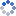加载中…MATLAB技术论坛
• 博客等级：• 博客积分：0
• 博客访问：590,608
• 关注人气：489
• 获赠金笔：0支
• 赠出金笔：0支
• 荣誉徽章：

## 【原创】MATLAB舍入误差和IEEE754标准深入分析

(2009-09-19 11:56:11)

### 杂谈

IEEE754-1985二进制浮点运算标准

MATLAB中所有数值都是使用64bit表示的。根据754-1985规定，第一位是符号指示位(S)，接下来的11位是指数部分(C)，最后称为尾数的52位为小数部分(F)，指数的基是2。

X64表示的区间范围

x64=0 10000000011 1011100100010000000000000000000000000000000000000000
y64=0 10000000011 1011100100001111111111111111111111111111111111111111
z64=0 10000000011 1011100100010000000000000000000000000000000000000001

[(y+x)/2, (x+z)/2)=
[15518507114430463/562949953421312, 15518507114430465/562949953421312)

xmin=2^(-1023)*(1+0.5^52)≈10^(-308)

xmax=2^1024*(2-0.5^52)≈10^308

X的精度范围求解

0